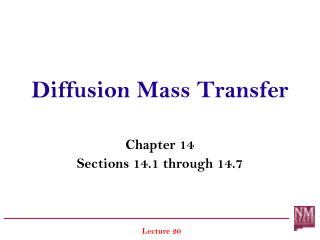DownloadDownload PresentationDiffusion Mass Transfer

# Diffusion Mass Transfer

Télécharger la présentation## Diffusion Mass Transfer

- - - - - - - - - - - - - - - - - - - - - - - - - - - E N D - - - - - - - - - - - - - - - - - - - - - - - - - - -

1. ### Diffusion Mass Transfer

Chapter 14 Sections 14.1 through 14.7 Lecture 20
2. Physical Origins and Rate Equations Mass Transfer in Nonstationary Media Conservation Equation and Diffusion through Stationary Media Diffusion and Concentrations at Interfaces Diffusion with Homogenous Reactions Transient Diffusion
3. 3. Boundary and Initial Conditions Henry’s Law: B.C.-1: at x=0, xA(0,t) = xA,s xA(0) = pA/H for gas–liquid interface where H [bar] is Henry’s constant, Table A.9 B.C.-1: at x=0, xA(0,t) = xA,s CA(0) = SpA for gas–solid interface where S is solubility of gas in a solid, [kmol/m3bar], S values can be found in Table A.10
4. Stationary Media with Catalytic Surface Reactions 1-D diffusion, Surface reaction, Steady-state, Planar medium: B.C.-1: B.C.-2:
5. Stationary Media with Catalytic Surface Reactions For stationary medium: Combined with B.C.-1 (1st order reaction): Solve the following ODE:
6. Stationary Media with Catalytic Surface Reactions Concentration distribution: At catalytic surface:
7. Stationary Media with Catalytic Surface Reactions At catalytic surface: Case-1: Reaction limited Case-2: Diffusion limited
8. Example 1 (Example 14.4) Helium gas is stored at 20C in a spherical container of fused silica (SiO2), which has a diameter of 0.2 m and wall thickness of 2 mm. If the container is charged to an initial pressure of 4 bar, what is the rate at which this pressure decreases with time?
9. Example 1 Known:Initial pressure of He in a spherical, fused silica container of prescribed diameter D and wall thickness L. Find: The rate of change of He pressure, dpA/dt Schematic:
10. Example 1 Assumptions: D>>L, diffusion can be approximated as 1-D through a plane wall Steady state diffusion at any instant through the silica wall Outside pressure of He is zero Ideal gas behavior for He Properties: Table A.8for He-fused silicaat 293K, DAB=0.4x10-13 m2/s Table A.10for He-fused silicaat 293K, S=0.45x10-3 kmol/m3bar
11. Example 1 Analysis: (1). Conversation of species (He) over the control volume: (2). Outflow due to diffusion :
12. Example 1 (3). Ideal gas assumption:
13. Example 1 or
14. Example 1 (4). From rate equation (v=0):
15. Example 1 (5). From steady-state assumption: (6). Henry’s Law: A,i= MA * S * pA,i A,1= MA * S * pA,1 =MASpA A,2= MA * S * pA,2 = 0
16. Example 1 (7). Final expression for dpA/dt A = D2, V= D3/6, A/V =6/D
17. Example 2 Hydrogen gas is maintained at 3 bar and 1 bar on opposite sides of a plastic membrane, which is 0.3 mm thick. The temperature is 25 C, and the binary diffusivity of H2 in the plastic is 8.7x10-8 m2/s. The solubility of H2 in the membrane is 1.5x10-3 kmol/m3bar. What is the mass diffusive flux of H2 through the membrane?
18. Example 2 Known:Pressure of H2 on opposite sides of a membrane. Find: The H2 mass diffusive flux (kg/sm2) Schematic:
19. Example 2 Assumptions: Steady state diffusion, 1-D conditions exist; Membrane is stationary nonreacting medium Analysis: From equation 14.49, for H2
20. Example 2 From the definition of solubility: On a mass basis:
21. 4. Diffusion with Homogeneous Reaction Homogeneous reaction is one that occurs within the medium. Heterogeneous reaction is one that occurs on the surface. For 1-D diffusion of species A through a planar medium of A &B with homogeneous reaction under steady-state conditions:
22. Diffusion with Homogeneous Reaction 1-D diffusion, Homogeneous reaction, Steady-state, Planar medium: Zero-order reaction: First-order reaction:
23. Diffusion with 1st Order Homogeneous Reaction 1-D diffusion, Homogeneous reaction , Steady-state, Planar medium: where:
24. Diffusion with 1st Order Homogeneous Reaction b.c.’s
25. Diffusion with 1st Order Homogeneous Reaction b.c.’s
26. Example 3 Consider the problem of O2 transfer from the interior lung cavity, across the lung tissue, to the network of blood vessels on the opposite side. The lung tissue (species B) may be approximately as a plane wall of thickness L. The inhalation process may be assumed to maintain a constant molar concentration CA(0) of O2 (Species A) in the tissue
27. Example 3 at its inner surface (x=0), and assimilation of O2 by the blood may be assumed to maintain a constant molar concentration CA(L) of O2 in the tissue at its outer surface (x=L). There is O2 consumption in the tissue due to metabolic processes, and the reaction is zero order, with =-k0. Obtain expression for the distribution of the O2 concentration in the tissue and the rate of assimilation of O2 by the blood per unit tissue surface.
28. Example 3 Known:Molar concentrations of oxygen at inner and outer surfaces of lung tissue. Volumetric rate of oxygen consumption within the tissue. Find: (a) Variation of oxygen molar concentration with position in the tissue, (b) Rate of oxygen transfer to the blood per unit tissue surface area. Schematic:
29. Example 3 Assumptions: (1) Steady-state conditions, (2) 1-D species transfer by diffusion through a plane wall, (3) Homogeneous, stationary medium with uniform total molar concentration and constant diffusion coefficient. Analysis:
30. Example 3 b.c.’s: x=0, CA(0)=CA,0;x=L, CA(L)=CA,L
31. Example 3 Lecture 20